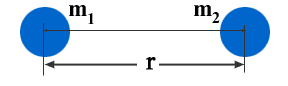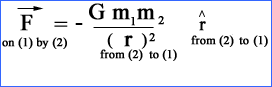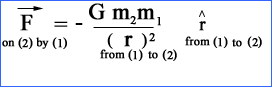# Gravitation

Gravitation
Every object in our universe attracts the other object with certain force towards its center. This force of attraction is known as GRAVITATIONAL FORCE and the phenomenon is called GRAVITATION. This is gravitational force which is responsible for the uniformity or regularity in our daily astronomical life. The whole system of the universe is in order only due to this force. Due to gravitation, the system of our universe is working uniformly and smoothly. The planets around the earth or around the sun moves in an orderly motion due to gravitation.

Newton's Law of GravitationIn order to explain the gravitational force between two bodies, Newton formulated a fundamental law known after his name i.e. “NEWTON’S LAW OF GRAVITATION”

Newton’s law of gravitation states thatevery object in the universe attracts the other object with a force and :

(1) The gravitational force of attraction between two bodies is directly proportional to the product of their masses.

F a m1 x m2 ——- (1)

(2) The gravitational force of attraction between two bodies is inversely proportional to the square of the distance between their centers.

F a 1/r2 ——— (2)

Mathematical Representation

Let us consider two bodies of masses m1 and m2 placed with their centres at distance of r from each other.

According to the Newton’s law of gravitation, the gravitational force of attraction between them is

F a m1 x m2 ——- (1)
F a 1/r1 ——— (2)
F a m1m2 /r1

Combining (1) and (2)

F = G m1m2/r1

Where is the constant of proportionality.

G = universal gravitational constant

Value of G:

G = 6.67 x 10-11 Nm2/kg2

Vectoral form of the law

The law of universal gravitation can also be expressed in vector form, which gives the magnitude as well as the direction of gravitational force.

The force exerted on body (1) by body (2) is always directed towards body (2) and acts along the line joining their centres
The displacement vector "r" from (2) to (1) is directed from body (2) to body (1), then,The force exerted on body (2) by body (1) is always directed towards body (1) and acts along the line joining their centres
The displacement vector "r" from (1) to (2) is directed from body (1) to body (2), then,The negative sign shows that the force of gravitation between two bodies is always an attractive force not a repulsive force.